Back to start Email meSimple intervals Neil Hawes Home Page
• Up to now, in all the sight-singing exercises you have done, notes have moved only one step at a time in a vertical direction:
• In other words, movement in single steps of the scale up or down.

• However, all real music has jumps as well as steps; so this is the next challenge, to be able to sight-sing simple jumps in pitch.

• An interval, when talking about musical notes, means the pitch gap between two notes.
• We measure the interval by the number of steps between the two notes on the stave:
• It's also the number of steps on the scale, since a scale occupies each consecutive position on the stave.
• The only catch is that we count inclusively, that is, the start and end positions are counted as well as the ones in between.

• Here's our trusty scale of C major on the treble clef stave, and a number of intervals are marked in: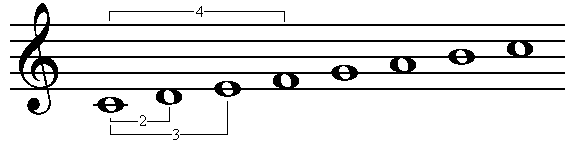• So we say there an interval of a fourth between the first and fourth notes of this scale (C and F).
• This doesn't mean there are four notes between the two - there are actually only two, but we count the start and end as well, making four.
• It makes more sense when you see that there is an interval of a second between the first two notes (C and D).

• So an interval of a second is what we have been calling a single step - you should be confident in singing these already.

• To sing an interval of a third, between the first note and the third note on the scale above, C to E, do the following:
• Sing the first three notes of the scale like this - try it on your own;
• Then do the same thing but leave out the middle note, sing it only in your head - like this - try it yourself;
• Now do it a little faster like this - and you are singing an interval of a third.

• To sing an interval of a fourth, between the first and the fourth notes on the scale above, C to F, do the same things:
• Sing the first four notes of the scale like this;
• Then leave out the two middle notes - like this;
• Now get faster until you can sing the two notes next to each other like this.
• It comes down to remembering the first note.

• Try the same for an interval of a fifth and sixth:
• They are not marked on the scale above, but it should be obvious what they are;
• Don't try a seventh - that is hard and anyway quite unusual in music.

• Not all jumps (intervals) start on the key note of the scale, you can measure the interval between any two notes on the stave or scale: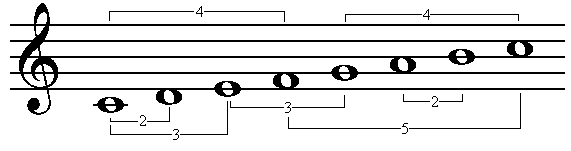• To sing, for example, the interval of a third that is between the third and fifth notes of the scale above (E to G) you need to:
• Sing the scale from the bottom, proceed through the lower note of the two and up to the higher note like this;
• Then leave out the notes below the lower note of the two like this;
• Then, as before, leave out the note(s) between the top and bottom ones like this;
• And finally, speed up and get the top and bottom notes together like this.

• The first step is most important - you have to start in the correct place in the scale:
• If the lower note of an interval is not the key note, you must not start singing a scale on it;
• If you do, you will not necessarily get the correct interval.
• When you get more confident, this will come naturally, from knowing the key note and scale of the piece you are singing at the time.

• If we combine two of the jumps we have already tried, from the first to the third, and then to the fifth, we get a triad:
• A major triad is the first, third, and fifth notes of the major scale;
• You should already be familiar with singing the triad, so you may want to go back and sing some again, now that you know more about what it is.
• The triad is very important in sight-singing - it occurs very fequently in almost all styles of music.

• So now let's try some sight-reading including simple intervals:
• This first one contains two easy examples of the interval of a third I have just described;
• Before you start, sing a scale to yourself (it doesn't really matter what note you start on) and count to four slowly;
• As you get to the note before the jump, try to "hear" the missing note in your head before you sing the higher one.
• Alternatively (or better, additionally), notice that three of the four notes in the first bar form the triad (C, E, G)
• So you can sing the triad and put in the extra second note (D).
• Also notice that the second and third bars are almost the same as the first and second bars, but up one note (this is called a sequence)
• If you are unsure about any of this, you can hear the whole thing here.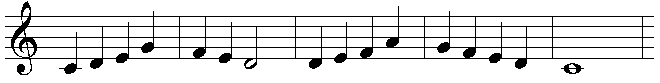• This one contains two jumps, one straight after the other (twice), but the first three notes are the triad, so should be easy to sing:
• Again, notice that the third bar is exactly the same as the first, but up one note.
• Patterns are very important in music.
• Also notice that it finishes on the key note an octave above the one it started on.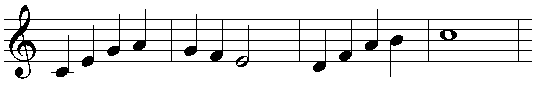hear it

• This one has downwards jumps of an interval of a third in the second and fourth bars:
• You can do these in perhaps three ways:
1. Either as above, by singing the missing note in your head;
2. Or remember the note that you sang three notes earlier;
3. Or notice that it is two notes of the triad;
• If you can do two or even three of these, you are much more likly to be sure of being correct! ("the belt and braces" technique).
• In bar 4, the notes are the same as in bar 2, so you might remember them from when you sang them 2 bars earlier.
• Memory is very important in sight-singing!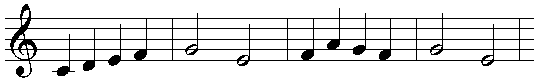hear it

• This one starts on a note which is not the key note and immediately jumps up an interval of a third:
• But these are the second and third note of the triad, you just have to make sure you start on the correct one.
• Notice that the second bar is the same as the first, but one note up.
• There is also a jump of an interval of a fourth in the third bar:
• This is a junp to the top note of the scale (the key note) you have practised this;
• So again you can use two techniques at once - this will help confirm that you get to the correct note.
• The jump to the very last note is to the same key note.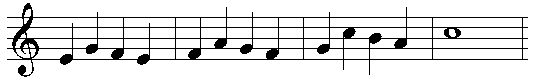hear it